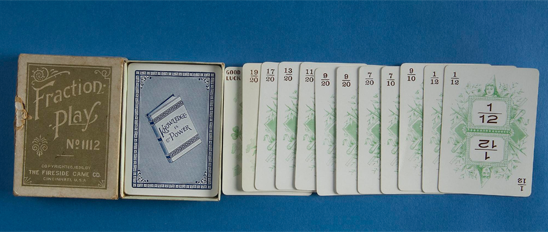# Arithmetic Teaching Apparatus -- Geometrical Models for Arithmetic Teaching

## Geometrical Models for Arithmetic TeachingStandard topics in arithmetic teaching are calculations of the area of plane figures and the volume of solids. To make these processes clearer to students, educators introduced a variety of geometric models. Models were also used to teach about fractions and to explain the process of taking cube roots.

## Contents